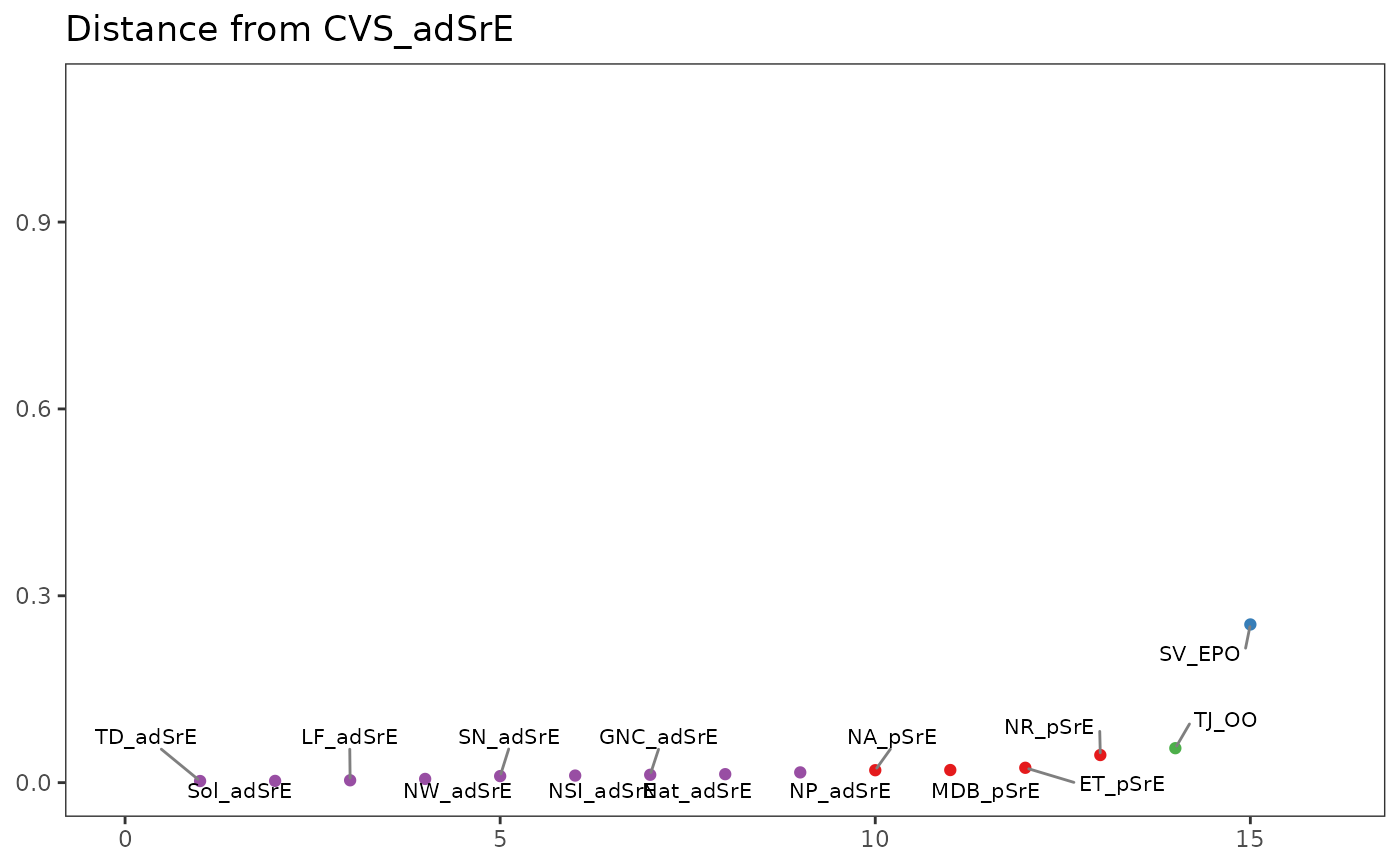This function plots the distance between a reference spectrum and all other spectra in a Spectra object. Distance can be defined in a number of ways (see Arguments).

plotSpectraDist(spectra, method = "pearson", ref = 1, labels = TRUE, ...)

## Arguments

spectra

An object of S3 class Spectra.

method

Character. Any method acceptable to rowDist.

ref

Integer. The spectrum to be used as a reference.

labels

Logical. Shall the points be labeled?

...

Parameters to be passed to the plotting routines. Applies to base graphics only.

## Value

The returned value depends on the graphics option selected (see GraphicsOptions).

base:

A data frame or list containing the data plotted. Assign the value and run str() or names() on it to see what it contains. Side effect is a plot.

ggplot2:

The plot is displayed, and a ggplot2 plot object is returned if the value is assigned. The plot can be modified in the usual ggplot2 manner. If you want the plotted values, you can access them via the base graphics mode.

To compare all spectra simultaneously in a heatmap, see sampleDist. Additional documentation at https://bryanhanson.github.io/ChemoSpec/

## Author

Bryan A. Hanson (DePauw University), Tejasvi Gupta.

## Examples


# This example assumes the graphics output is set to ggplot2 (see ?GraphicsOptions).
library("ggplot2")
data(SrE.NMR)
txt1 <- paste("Distance from", SrE.NMR$names) txt2 <- paste("Rank Distance from", SrE.NMR$names)